Examples

Chapter 5 Class 12 Continuity and Differentiability
Serial order wise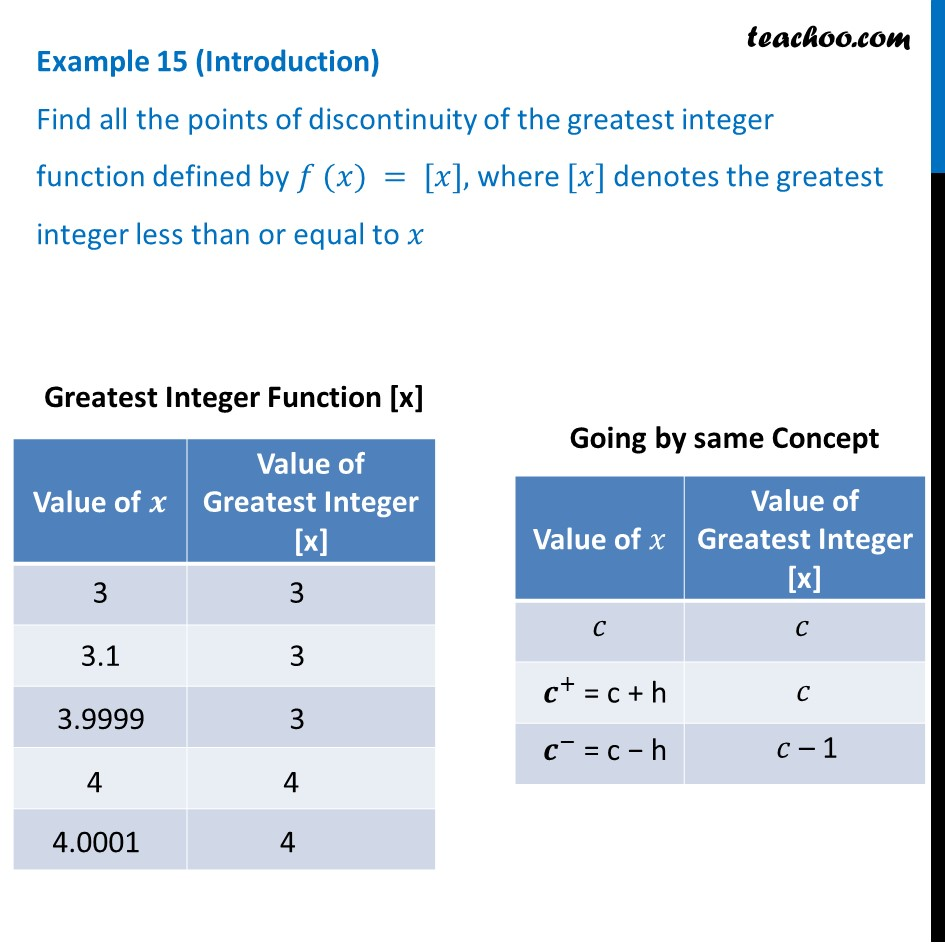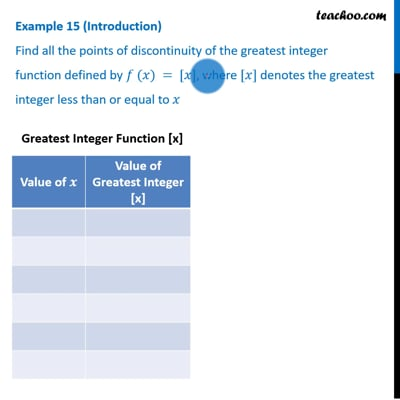This video is only available for Teachoo black users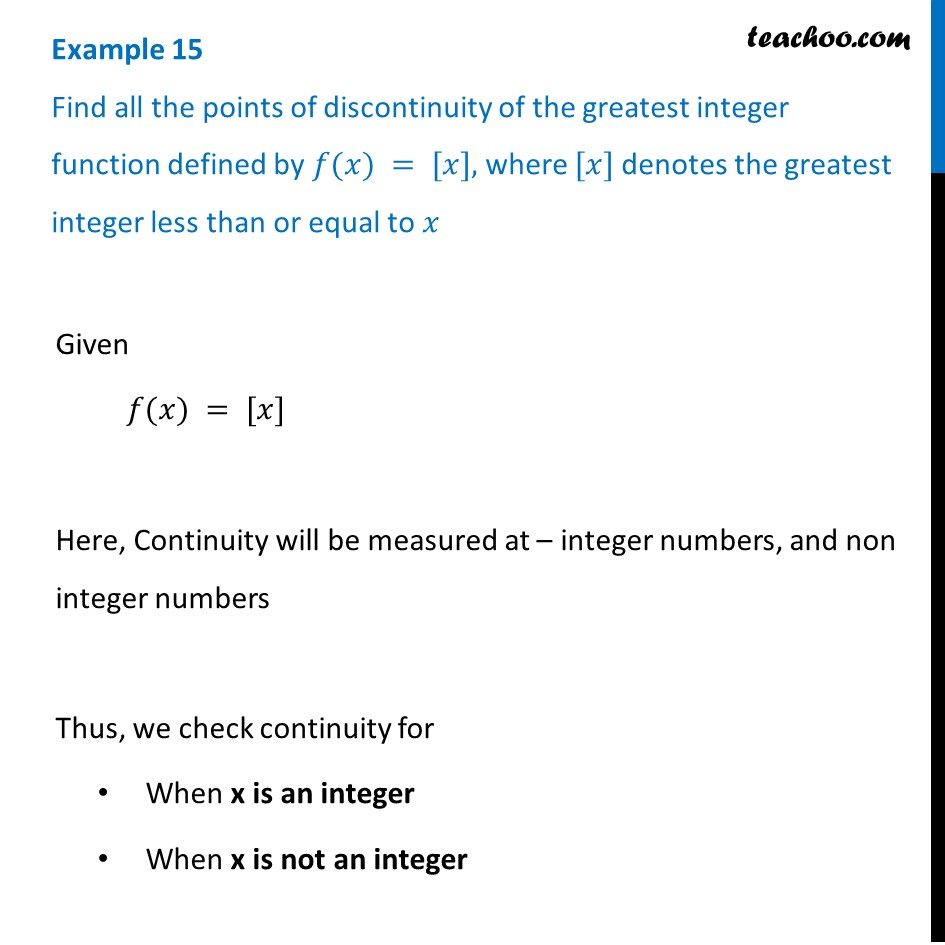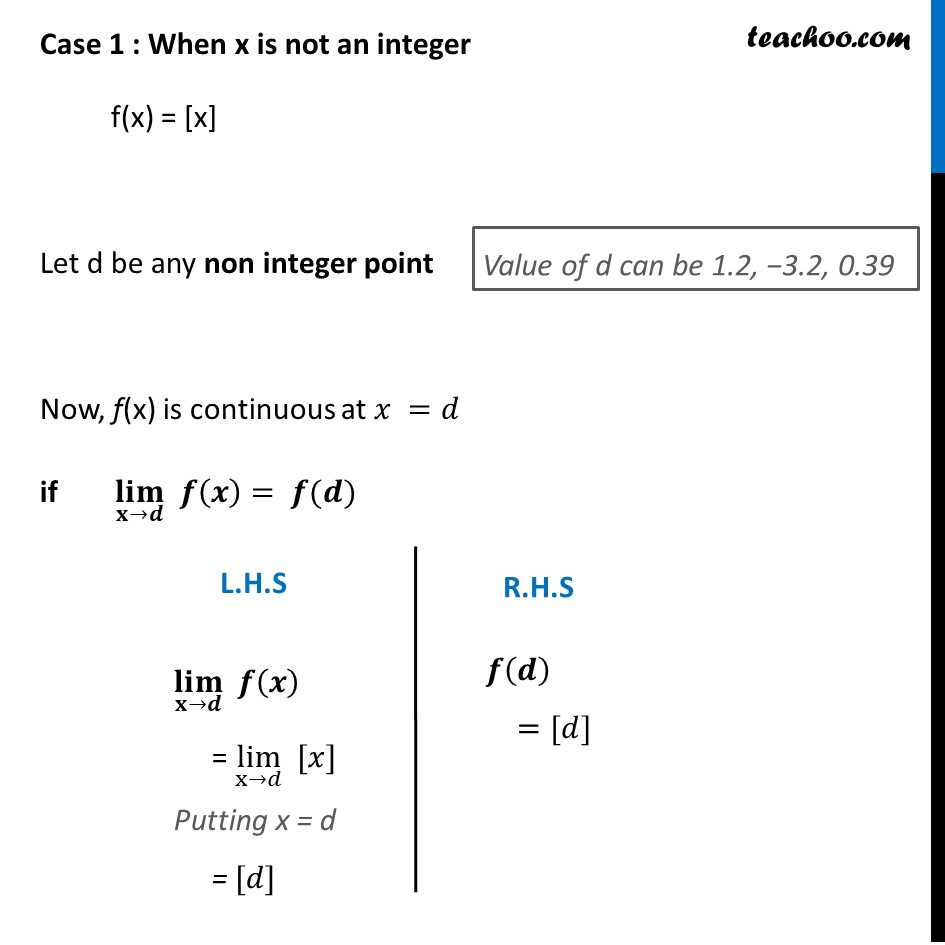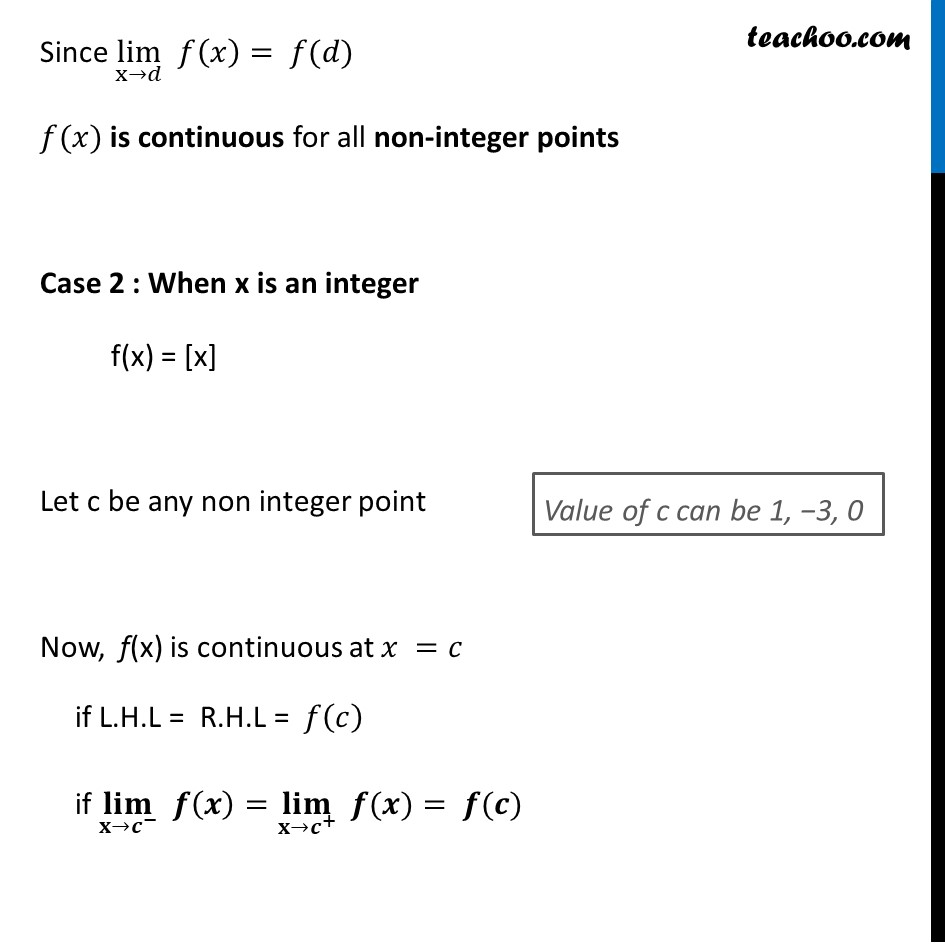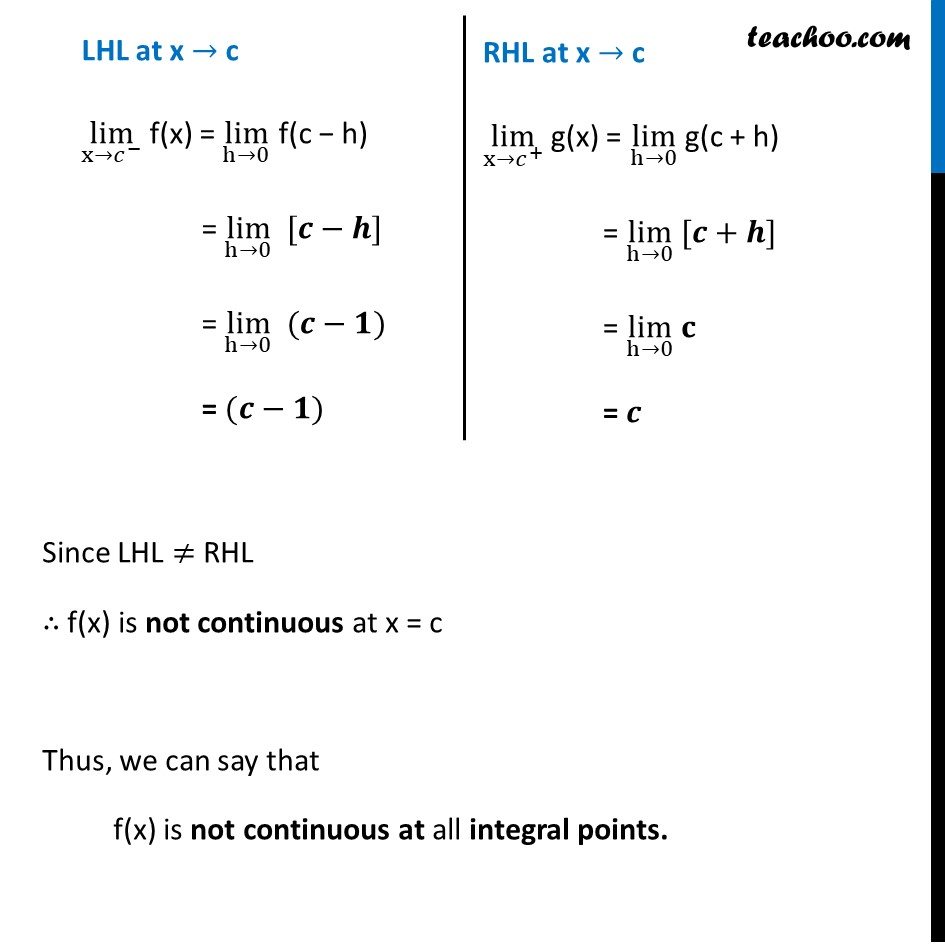Solve all your doubts with Teachoo Black (new monthly pack available now!)

### Transcript

Example 15 (Introduction) Find all the points of discontinuity of the greatest integer function defined by 𝑓 (𝑥) = [𝑥], where [𝑥] denotes the greatest integer less than or equal to 𝑥Greatest Integer Function [x] Going by same Concept Example 15 Find all the points of discontinuity of the greatest integer function defined by 𝑓(𝑥) = [𝑥], where [𝑥] denotes the greatest integer less than or equal to 𝑥Given 𝑓(𝑥) = [𝑥] Here, Continuity will be measured at – integer numbers, and non integer numbers Thus, we check continuity for When x is an integer When x is not an integer Case 1 : When x is not an integer f(x) = [x] Let d be any non integer point Now, f(x) is continuous at 𝑥 =𝑑 if (𝐥𝐢𝐦)┬(𝐱→𝒅) 𝒇(𝒙)= 𝒇(𝒅) Value of d can be 1.2, −3.2, 0.39 (𝐥𝐢𝐦)┬(𝐱→𝒅) 𝒇(𝒙) = lim┬(x→𝑑) [𝑥] Putting x = d = [𝑑] 𝒇(𝒅) =[𝑑] Since lim┬(x→𝑑) 𝑓(𝑥)= 𝑓(𝑑) 𝑓(𝑥) is continuous for all non-integer points Case 2 : When x is an integer f(x) = [x] Let c be any non integer point Now, f(x) is continuous at 𝑥 =𝑐 if L.H.L = R.H.L = 𝑓(𝑐) if (𝐥𝐢𝐦)┬(𝐱→𝒄^− ) 𝒇(𝒙)=(𝐥𝐢𝐦)┬(𝐱→𝒄^+ ) " " 𝒇(𝒙)= 𝒇(𝒄) Value of c can be 1, −3, 0 LHL at x → c lim┬(x→𝑐^− ) f(x) = lim┬(h→0) f(c − h) = lim┬(h→0) [𝒄−𝒉] = lim┬(h→0) (𝒄−𝟏) = (𝒄−𝟏) RHL at x → c lim┬(x→𝑐^+ ) g(x) = lim┬(h→0) g(c + h) = lim┬(h→0) [𝒄+𝒉] = lim┬(h→0) 𝐜 = 𝒄 Since LHL ≠ RHL ∴ f(x) is not continuous at x = c Thus, we can say that f(x) is not continuous at all integral points.